Get instant live expert help with Excel or Google Sheets“My Excelchat expert helped me in less than 20 minutes, saving me what would have been 5 hours of work!”

#### Post your problem and you’ll get Expert help in seconds.

Your message must be at least 40 characters
Our professional Expert are available now. Your privacy is guaranteed.

# Get middle name from full name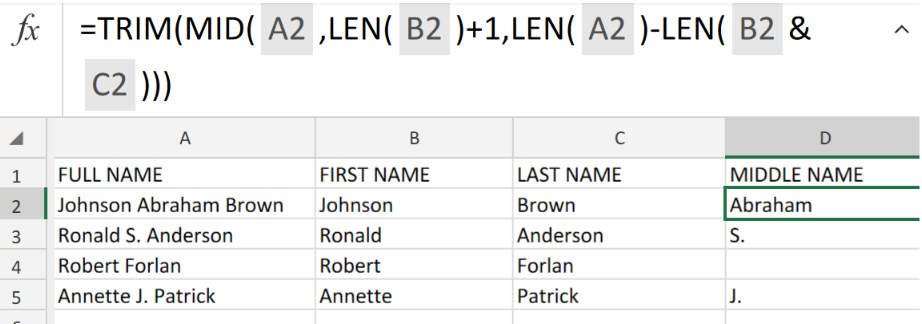Figure 1. of Get Middle Name from Full Name in Excel.

In the event that we are required to obtain a middle name from a full name, we are going to use the LEN and MID Functions as well as the TRIM Function. This tutorial will step through the process

## Generic Formula

`=TRIM(MID(name,LEN(first)+1,LEN(name)-LEN(first&last)))`

## How to Use the Excel LEN, MID and TRIM Functions in Excel.

We can achieve this by following three simple steps!

1. Let us assume that we have a person’s full name in our Excel sheet column A, the first name in another column B, the last name in a third column C. See example illustrated below;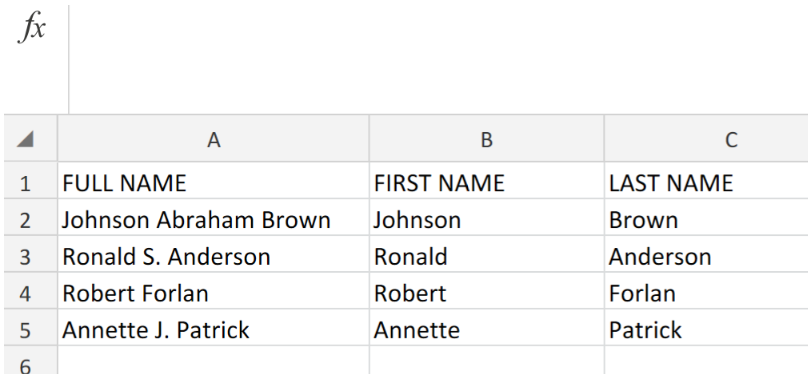Figure 2. of Full name in Excel sheet.

1. Label the next column D as “MIDDLE NAME”. This is where Excel will return the Middle Name data value. The formula syntax which we will enter into the formula bar for cell D2 in the example illustrated below, is as follows;

`=TRIM(MID(A2,LEN(B2)+1,LEN(A2)-LEN(B2&C2)))`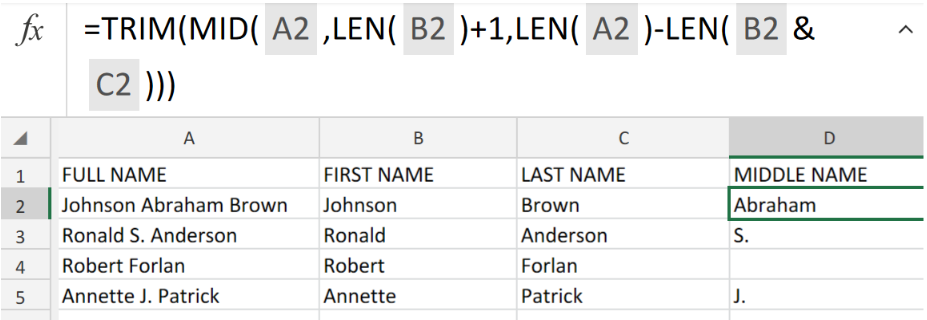Figure 3. of LEN, MID and TRIM Functions in Excel.

The Excel MID Function will start by extracting text from our full name text in column A, beginning from 1 character which comes after the first name. The Excel TRIM Function then begins the operation of clearing out any extra spaces or unwanted characters.

1. Copy the formula in cell D2 of our worksheet illustration into the other cells in column D to get the desired results.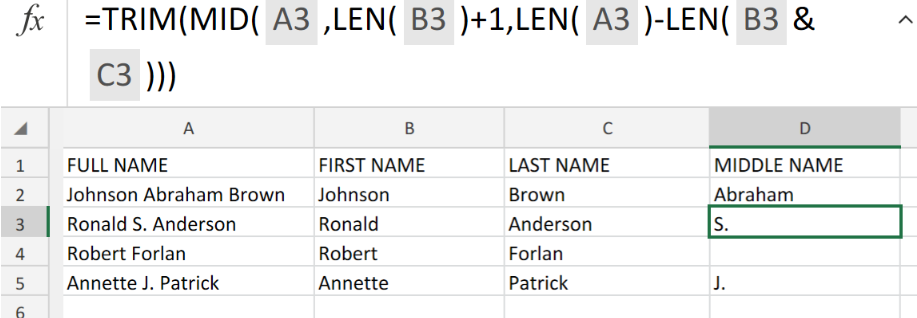Figure 4. of LEN, MID and TRIM Functions in Excel.

## Note

• If there is an existing middle name, the Excel MID Function will extract this middle name then the Excel TRIM Function proceeds to eliminate any extra space.
• In the event that we have more than one existing middle name, the Excel MID Function will extract all these middle names and then the Excel TRIM Function proceeds to eliminate any extra space.
• If there happens to be no existing middle name, the Excel MID Function will automatically return a blank/space character which the Excel TRIM Function will proceed to eliminate.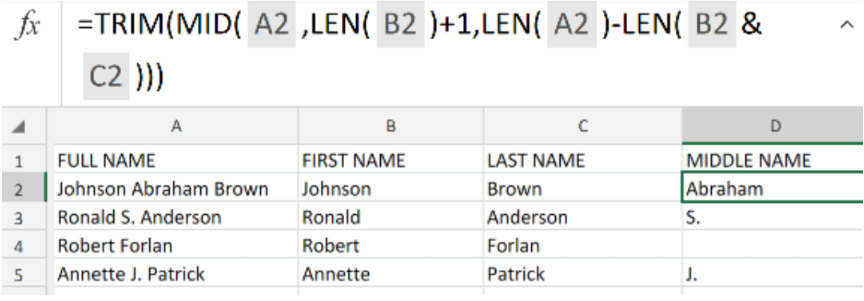Figure 5. of Final Result.

## Instant Connection to an Expert through our Excelchat Service

Our live Excelchat Service is here for you. We have Excel Experts available 24/7 to answer any Excel questions you may have. Guaranteed connection within 30 seconds and a customized solution for you within 20 minutes.

### Did this post not answer your question? Get a solution from connecting with the expert.Another blog reader asked this question today on Excelchat:
Solution examplesI need a formula .... i need to decrease the premium an employee pays by 10% each year for ages 65-75. So when they are 65 it decreases 10%, age 66 it decreases 20% and so on. I tried =IF(OR(\$D2+1<65,\$D2+1=66,\$D2+2=77, ...... ),J2,J2-(J2*0.1),J2(J2*0.2) .... ) D2 being the current age of the employee, with each column being year 1, year 2, etc. However, excel will not allow me to do to IF D2+1=66, til age 75. It's too long. I know there has to be a better way! HELP!
Solved by F. F. in 60 minsCan you re edit this formula for me =IFERROR(IF(LEN(MID("RHS",1,FIND("RHS",B4,1)))=1,LEFT(B4,3),MID("RHS",1,FIND("RHS",B4,1))),MID("LHS",1,FIND("LHS",B4,1)))
Solved by S. L. in 40 minsIf I have a sheet that pulls data from another sheet. How can I compare if the right data is filled in, after the fact?
Solved by Z. Y. in 43 minsI have text and number in multiple cells (Ex: Cell 1: 4S, Cell 2: 3.5A, Cell 3: 8O) and want to sum the number values only (Ex: 4 + 3.5 + 8). How can I do this?
Solved by O. A. in 60 minsa date formula that results in showing the date as 29/12/00 (date format as dd/mm/yy) - use column BA to calculate 364 days (same date format) in column BS, if BA blank, then calculate 364 days using column AX. Results for column BA are correct. However, if column BA is empty, then it should calculate using column AX, which has data, but the result is always 29/12/00, regardless of the date in column AX. I have used this formula with success in another workbook, but this file wont work! Formula: =IF(AND(LEN(\$BA74)=0,LEN(\$AX74)=0),"",IF(LEN(\$BA74)=0,DATE(YEAR(\$AX74),MONTH(\$AX74),DAY(\$AX74)+364),DATE(YEAR(\$BA74),MONTH(\$BA74),DAY(\$BA74)+364)))
Solved by A. L. in 60 mins## Subscribe to Excelchat.coAnother blog reader asked this question today on Excelchat: